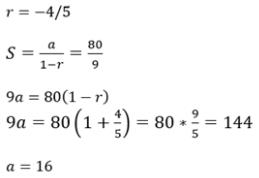Courses

# Test: Sum To Infinity Of A GP

## 5 Questions MCQ Test Mathematics (Maths) Class 11 | Test: Sum To Infinity Of A GP

Description
This mock test of Test: Sum To Infinity Of A GP for JEE helps you for every JEE entrance exam. This contains 5 Multiple Choice Questions for JEE Test: Sum To Infinity Of A GP (mcq) to study with solutions a complete question bank. The solved questions answers in this Test: Sum To Infinity Of A GP quiz give you a good mix of easy questions and tough questions. JEE students definitely take this Test: Sum To Infinity Of A GP exercise for a better result in the exam. You can find other Test: Sum To Infinity Of A GP extra questions, long questions & short questions for JEE on EduRev as well by searching above.
QUESTION: 1

### 61/2.61/4.61/8.- - - - -∞ = ________

Solution: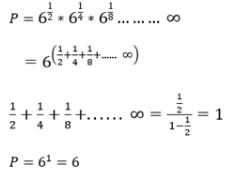QUESTION: 2

### The sum of the series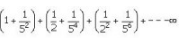Solution: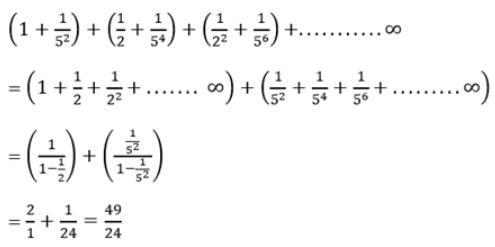QUESTION: 3

###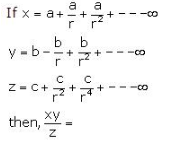Solution:
QUESTION: 4

2¹ + 2² +2³ +….+2n =

Solution:
QUESTION: 5

The common ratio of a G.P. is -4/5 and the sum to infinity is 80/9, then its first term is

Solution: